# Fractals

A fractal is a geometric figure that often can be generated from a pattern repeated recursively an infinite number of times (Fig. 15.17). The figure is modified by applying the pattern to each segment of the original figure. Real geometric figures do not have their patterns repeated an infinite number of times, but after several repetitions appear as they would if repeated infinitely. We will look at a few such approximations in this section. [Note: We will refer to our geometric figures as fractals, even though they are approximations.] Although these figures had been studied before the 20th century, it was the Polish mathematician Benoit Mandelbrot who introduced the term "fractal" in the 1970s, along with the specifics of how a fractal is created and the practical applications of fractals. Mandelbrot's fractal geometry provides mathematical models for many complex forms found in nature, such as mountains, clouds and coastlines. Fractals have many uses in mathematics and science. Fractals can be used to better understand systems or patterns that appear in nature (e.g., ecosystems), in the human body (e.g., in the folds of the brain), or in the universe (e.g., galaxy clusters). Drawing fractals has become a popular art form. Fractals have a self-similar propertywhen subdivided into parts, each resembles a reduced-size copy of the whole. Many fractals yield an exact copy of the original when a portion of the original image is magnifiedsuch a fractal is said to be strictly self similar. Links are provided in Section 15.12 for various Web sites that discuss and demonstrate fractals.

Figure 15.17. Koch Curve fractal.As an example, let us look at a popular strictly self-similar fractal known as the Koch Curve (Fig. 15.17). This fractal is formed by removing the middle third of each line in the drawing and replacing it with two lines that form a point, such that if the middle third of the original line remained, an equilateral triangle would be formed. Formulas for creating fractals often involve removing all or part of the previous fractal image. This pattern has already been determined for this fractalin this section we focus not on how to determine what formulas are needed for a specific fractal, but how to use those formulas within a recursive solution. Most formulas can be used to create fractals, but not all fractals will resemble objects in nature.

We start with a straight line (Fig. 15.17, Part a) and apply the pattern, creating a triangle from the middle third (Fig. 15.17, Part b). We then apply the pattern again to each straight line, resulting in Fig. 15.17, Part c. Each time the pattern is applied, we say that the fractal is at a new level, or depth (sometimes the term order is also used). Fractals can be displayed at many levelsfor instance, a fractal at level 3 has had three iterations of the pattern applied (Fig. 15.17, Part d). After only a few iterations, this fractal begins to look the same, like a portion of a snowflake (Fig. 15.17, Parts e and f). The applications of the pattern are now too small for the human eye to see a difference. Since this is a strictly self-similar fractal, each portion of the fractal contains an exact copy of the fractal. In Part f of Fig. 15.17, for example, we have highlighted a portion of the fractal with a dashed red box. If the image in this box were increased in size, it would look exactly like the entire fractal of Part f.

There is a similar fractal, the Koch Snowflake, which is the same as the Koch Curve but begins with a triangle rather than a line. The same pattern is applied to each side of the triangle, resulting in an image that looks like an enclosed snowflake. We have chosen to focus on the Koch Curve for simplicity. We will be moving on shortly to another fractal, but if the reader wishes to learn more about the Koch Curve and Koch Snowflake, see the links in Section 15.12.

We now demonstrate the use of recursion to draw fractals by writing a program to create a strictly self-similar fractal. We call this the "Lo fractal," named for Sin Han Lo, a Deitel & Associates colleague who created it. The fractal will eventually resemble one-half of a feather (see the outputs in Fig. 15.24). The base case, or fractal level of 0, begins as a line between two points, A and B (Fig. 15.18). To create the next higher level, we find the midpoint (C) of the line. To calculate the location of point C, use the following formula: [Note: The x and y to the left of each letter refer to the x-coordinate and y-coordinate of that point, respectively. For instance, xA refers to the x-coordinate of point A, while yC refers to the y-coordinate of point C. In our diagrams we denote the point by its letter, followed by two numbers representing the x- and y-coordinates.]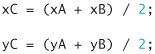Figure 15.18'. "Lo fractal" at level 0.

(This item is displayed on page 766 in the print version)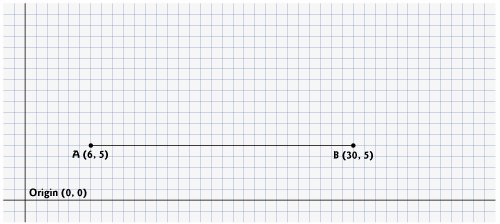To create this fractal, we also must find a point D that lies left of segment AC and creates an isosceles right triangle ADC. To calculate the location of point D, use the following formulas: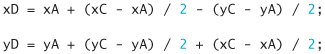We continue the example in Fig. 15.18 by moving on to the next level (from level 0 to level 1) as follows: First, add points C and D (as in Fig. 15.19). Then, remove the original line and add segments DA, DC and DB. The remaining lines curve at an angle, causing our fractal to look like a feather. For the next level of the fractal, this algorithm is repeated on each of the three lines in level 1. For each line, the formulas above are applied, where the former point D is now considered to be point A, while the other end of each line is considered to be point B. Figure 15.20 contains the line from level 0 (now a dashed line) and the three added lines from level 1. We have changed point D to be point A, and the original points A, C and B to B1, B2 and B3 (the numbers are used to differentiate the various points). The preceding formulas have been used to find the new points C and D on each line. These points are also numbered 13 to keep track of which point is associated with each line. The points C1 and D1, for instance, represent points C and D associated with the line formed from points A to B1. To achieve level 2, the three lines in Fig. 15.20 are removed and replaced with new lines from the C and D points just added. Figure 15.21 shows the new lines (the lines from level 2 are shown as dashed lines for your convenience). Figure 15.22 shows level 2 without the dashed lines from level 1. Once this process has been repeated several times, the fractal created will begin to look like one-half of a feather, as shown in the output of Fig. 15.24. We will discuss the code for this application shortly.

Figure 15.19. Determining points C and D for level 1 of "Lo fractal."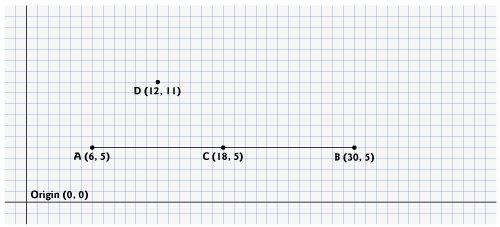Figure 15.20. "Lo fractal" at level 1, with C and D points determined for level 2. [Note: The fractal at level 0 is included as a dashed line as a reminder of where the line was located in relation to the current fractal.

(This item is displayed on page 767 in the print version)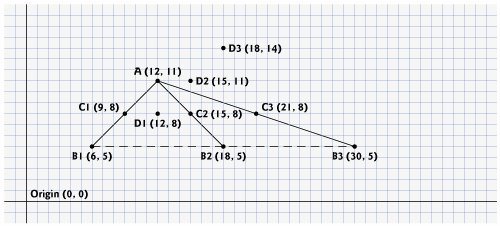Figure 15.21. "Lo fractal" at level 2, with dashed lines from level 1 provided.

(This item is displayed on page 767 in the print version)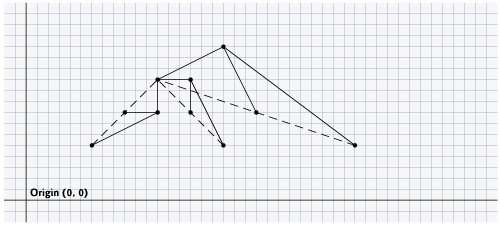Figure 15.22. "Lo fractal" at level 2.

(This item is displayed on page 768 in the print version)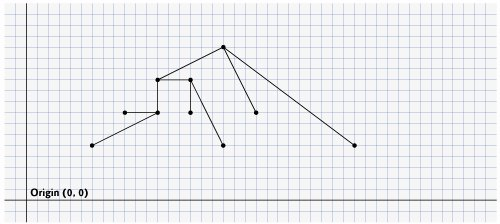Figure 15.23. Demonstrating the fractal user interface.

(This item is displayed on pages 768 - 770 in the print version)

 ``` 1 // Fig. 15.23: Fractal.java 2 // Demonstrates user interface for drawing a fractal. 3 import java.awt.Color; 4 import java.awt.FlowLayout; 5 import java.awt.event.ActionEvent; 6 import java.awt.event.ActionListener; 7 import javax.swing.JFrame; 8 import javax.swing.JButton; 9 import javax.swing.JLabel; 10 import javax.swing.JPanel; 11 import javax.swing.JColorChooser; 12 13 public class Fractal extends JFrame 14 { 15 private final int WIDTH = 400; // define width of GUI 16 private final int HEIGHT = 480; // define height of GUI 17 private final int MIN_LEVEL = 0; 18 private Color color = Color.BLUE; 19 20 private JButton changeColorJButton, increaseLevelJButton, 21 decreaseLevelJButton; 22 private JLabel levelJLabel; 23 private FractalJPanel drawSpace; 24 private JPanel mainJPanel, controlJPanel; 25 26 // set up GUI 27 public Fractal() 28 { 29 super( "Fractal" ); 30 31 // set up control panel 32 controlJPanel = new JPanel(); 33 controlJPanel.setLayout( new FlowLayout() ); 34 35 // set up color button and register listener 36 changeColorJButton = new JButton( "Color" ); 37 controlJPanel.add( changeColorJButton ); 38 changeColorJButton.addActionListener( 39 new ActionListener() // anonymous inner class 40 { 41 // process changeColorJButton event 42 public void actionPerformed( ActionEvent event ) 43 { 44 color = JColorChooser.showDialog( 45 Fractal.this, "Choose a color", color ); 46 47 // set default color, if no color is returned 48 if ( color == null ) 49 color = Color.BLUE; 50 51 drawSpace.setColor( color ); 52 } // end method actionPerformed 53 } // end anonymous inner class 54 ); // end addActionListener 55 56 // set up decrease level button to add to control panel and 57 // register listener 58 decreaseLevelJButton = new JButton( "Decrease Level" ); 59 controlJPanel.add( decreaseLevelJButton ); 60 decreaseLevelJButton.addActionListener( 61 new ActionListener() // anonymous inner class 62 { 63 // process decreaseLevelJButton event 64 public void actionPerformed( ActionEvent event ) 65 { 66 int level = drawSpace.getLevel(); 67 level--; // decrease level by one 68 69 // modify level if possible 70 if ( level >= MIN_LEVEL ) 71 { 72 levelJLabel.setText( "Level: " + level ); 73 drawSpace.setLevel( level ); 74 repaint(); 75 } // end if 76 } // end method actionPerformed 77 } // end anonymous inner class 78 ); // end addActionListener 79 80 // set up increase level button to add to control panel 81 // and register listener 82 increaseLevelJButton = new JButton( "Increase Level" ); 83 controlJPanel.add( increaseLevelJButton ); 84 increaseLevelJButton.addActionListener( 85 new ActionListener() // anonymous inner class 86 { 87 // process increaseLevelJButton event 88 public void actionPerformed( ActionEvent event ) 89 { 90 int level = drawSpace.getLevel(); 91 level++; // increase level by one 92 93 // modify level if possible 94 if ( level >= MIN_LEVEL ) 95 { 96 levelJLabel.setText( "Level: " + level ); 97 drawSpace.setLevel( level ); 98 repaint(); 99 } // end if 100 } // end method actionPerformed 101 } // end anonymous inner class 102 ); // end addActionListener 103 104 // set up levelJLabel to add to controlJPanel 105 levelJLabel = new JLabel( "Level: 0" ); 106 controlJPanel.add( levelJLabel ); 107 108 drawSpace = new FractalJPanel( 0 ); 109 110 // create mainJPanel to contain controlJPanel and drawSpace 111 mainJPanel = new JPanel(); 112 mainJPanel.add( controlJPanel ); 113 mainJPanel.add( drawSpace ); 114 115 add( mainJPanel ); // add JPanel to JFrame 116 117 setSize( WIDTH, HEIGHT ); // set size of JFrame 118 setVisible( true ); // display JFrame 119 } // end Fractal constructor 120 121 public static void main( String args[] ) 122 { 123 Fractal demo = new Fractal(); 124 demo.setDefaultCloseOperation( JFrame.EXIT_ON_CLOSE ); 125 } // end main 126 } // end class Fractal ```

Figure 15.24. Drawing "Lo fractal" using recursion.

(This item is displayed on pages 771 - 773 in the print version)

``` 1 // Fig. 15.24: FractalJPanel.java
2 // FractalJPanel demonstrates recursive drawing of a fractal.
3 import java.awt.Graphics;
4 import java.awt.Color;
5 import java.awt.Dimension;
6 import javax.swing.JPanel;
7
8 public class FractalJPanel extends JPanel
9 {
10 private Color color; // stores color used to draw fractal
11 private int level; // stores current level of fractal
12
13 private final int WIDTH = 400; // defines width of JPanel
14 private final int HEIGHT = 400; // defines height of JPanel
15
16 // set the initial fractal level to the value specified
17 // and set up JPanel specifications
18 public FractalJPanel( int currentLevel )
19 {
20 color = Color.BLUE; // initialize drawing color to blue
21 level = currentLevel; // set initial fractal level
22 setBackground( Color.WHITE );
23 setPreferredSize( new Dimension( WIDTH, HEIGHT ) );
24 } // end FractalJPanel constructor
25
26 // draw fractal recursively
27 public void drawFractal( int level, int xA, int yA, int xB,
28  int yB, Graphics g )
29 {
30  // base case: draw a line connecting two given points
31  if ( level == 0 )
32  g.drawLine( xA, yA, xB, yB );
33  else // recursion step: determine new points, draw next level
34  {
35  // calculate midpoint between (xA, yA) and (xB, yB)
36  int xC = ( xA + xB ) / 2;
37  int yC = ( yA + yB ) / 2;
38
39  // calculate the fourth point (xD, yD) which forms an
40  // isosceles right triangle between (xA, yA) and (xC, yC)
41  // where the right angle is at (xD, yD)
42  int xD = xA + ( xC - xA ) / 2 - ( yC - yA ) / 2;
43  int yD = yA + ( yC - yA ) / 2 + ( xC - xA ) / 2;
44
45  // recursively draw the Fractal
46  drawFractal( level - 1, xD, yD, xA, yA, g );
47  drawFractal( level - 1, xD, yD, xC, yC, g );
48  drawFractal( level - 1, xD, yD, xB, yB, g );
49  } // end else
50 } // end method drawFractal
51
52 // start the drawing of fractal
53 public void paintComponent( Graphics g )
54 {
55 super .paintComponent( g );
56
57 // draw fractal pattern
58 g.setColor( color );
59 drawFractal( level, 100, 90, 290, 200, g );
60 } // end method paintComponent
61
62 // set the drawing color to c
63 public void setColor( Color c )
64 {
65 color = c;
66 } // end method setColor
67
68 // set the new level of recursion
69 public void setLevel( int currentLevel )
70 {
71 level = currentLevel;
72 } // end method setLevel
73
74 // returns level of recursion
75 public int getLevel()
76 {
77 return level;
78 } // end method getLevel
79 } // end class FractalJPanel
```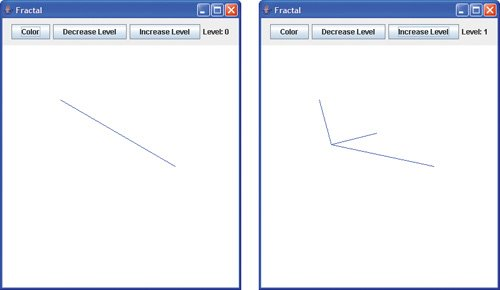The application in Fig. 15.23 defines the user interface for drawing this fractal (shown at the end of Fig. 15.24). The interface consists of three buttonsone for the user to change the color of the fractal, one to increase the level of recursion and one to decrease the level of recursion. A JLabel will keep track of the current level of recursion, which is modified by calling method setLevel, to be discussed shortly. Lines 1516 specify constants WIDTH and HEIGHT to be 400 and 480 respectively for the size of the JFrame. The default color to draw the fractal will be blue (line 18). The user triggers an ActionEvent by clicking the Color button. The event handler for this button is registered in lines 3854. The method actionPerformed displays a JColorChooser. This dialog returns the selected Color object or blue (if the user presses Cancel or closes the dialog without pressing OK). Line 51 calls the setColor method in class FractalJPanel to update the color.

The event handler for the Decrease Level button is registered in lines 6078. In method actionPerformed, lines 6667 retrieve the current level of recursion and decrement it by 1. Line 70 checks to make sure that the level is greater than or equal to 0 (MIN_LEVEL). This is because the fractal is not defined for any recursion level lower than 0. The program allows the user to go up to any desired level, but it is important to note that at a certain point (level 10 and higher in this example) the rendering of the fractal becomes increasingly slower, as there is a lot of detail to be drawn. Lines 7274 reset the level label to reflect the changethe new level is set and the repaint method is called to update the image to show the fractal corresponding to the new level.

The Increase Level JButton works the same way as the Decrease Level JButton, except that the level is incremented rather than decremented to show more details of the fractal (lines 9091). When the application is first executed, the level will be set to 0, which will display a blue line between two points that were specified in the FractalJPanel class.

The FractalJPanel class in Fig. 15.24 specifies the dimensions of the drawing JPanel to be 400 by 400 (lines 1314). The FractalJPanel constructor (lines 1824) takes the current level as a parameter and assigns it to its instance variable level. Instance variable color is set to the default color blue. Lines 2223 change the background color of the JPanel to be white (for visibility of the colors used to draw the fractal), and set the new dimensions of the JPanel where the fractal will be drawn.

Lines 2750 define the recursive method that creates the fractal. This method takes six parameters: the level, four integers that specify the x and y coordinates of two points, and the Graphics object g. The base case for this method (line 31) occurs when level equals 0, at which time a line will be drawn between the two points given as parameters. Lines 3643 calculate (xC, yC), the midpoint between (xA, yA) and (xB, yB), and (xD, yD), the point that creates a right isosceles triangle with (xA, yA) and (xC, yC). Lines 4648 make three recursive calls on three different sets of points.

In method paintComponent, line 59 makes the first call to method drawFractal to start the drawing. This method call is not recursive, but all subsequent calls to drawFractal performed from the body of drawFractal are. Since the lines will not be drawn until the base case is reached, the distance between two points decreases on each recursive call. As the level of recursion increases, the fractal becomes smoother and more detailed. The shape of this fractal stabilizes as the level approaches 11. Fractals will stabilize at different levels based on the shape and size of the fractal.

Fig. 15.24 shows the development of the fractal from level zero to six. The last image shows the defining shape of the fractal at level 11. If we focus on one of the arms of this fractal, it would be identical to the whole image. This property defines the fractal to be strictly self-similar. See Section 15.12 for further resources on fractals.Java How to Program (6th Edition) (How to Program (Deitel))
ISBN: 0131483986
EAN: 2147483647
Year: 2003
Pages: 615